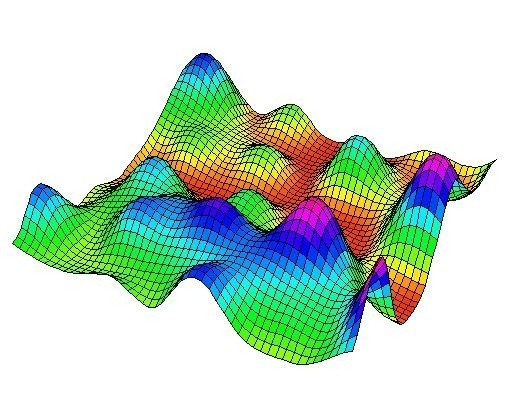2019 iT 邦幫忙鐵人賽DAY 27
0

Tensorflow 實作 MLP 影像辨識

Moore 先跟大家分享自己看一篇文章談到為何參數初始化很重要，他先把印出來的圖片秀給大家看Moore 請大家用 colab 的資源來做 Tensorflow 的練習，以下需要 Google 帳號來取得計算資源。

import tensorflow as tf

X = tf.placeholder(tf.float32, [None, 28, 28, 1])
W = tf.Variable(tf.zeros([784, 10]))
b = tf.Variable(tf.zeros())

init = tf.initialize_all_variables()

placeholder ： 置放資料集，不會去更新其值
Variable：參數放於此類型，持續更新用

Y = tf.nn.softmax(tf.matmul(tf.reshape(X, [-1, 784]), W) + b)
Y_ = tf.placeholder(tf.float32, [None, 10])
cross_entropy = -tf.reduce_sum(Y_ * tf.log(Y))

is_correct = tf.equal(tf.argmax(Y,1), tf.argmax(Y_,1))
accuracy = tf.reduce_mean(tf.cast(is_correct, tf.float32))
train_step = optimizer.minimize(cross_entropy)

sess = tf.Session()
sess.run(init)

for i in range(1000):
# load batch of images and correct answers
batch_X, batch_Y = mnist.train.next_batch(100)
train_data={X: batch_X, Y_: batch_Y}

# train
sess.run(train_step, feed_dict=train_data)

Fields 嘗試在 colab 的『代碼執行程序』選單選擇了 『更改運行類型』為 GPU，發現執行時間居然比 CPU 費時：再改為傳奇的Machine Learning 計算加速神器 TPU，時間還是更長。與一般刻板印象這些GPU, TPU加速器會讓計算速度加快，他們兩個花費時間更長。

Pete 幫大家查了一下，可以用這樣的程式碼取得哪些計算資源可利用

from tensorflow.python.client import device_lib
local_device_protos = device_lib.list_local_devices()
local_device_protos

incarnation: 15244691534819004833
physical_device_desc: "device: XLA_GPU device", name: "/device:GPU:0"
device_type: "GPU"
memory_limit: 11281553818
locality {
bus_id: 1
}
}
incarnation: 15257351585813503532
physical_device_desc: "device: 0, name: Tesla K80, pci bus id: 0000:00:04.0, compute capability: 3.7"]